Pandas dataframe, German vocabulary – select words by matching a few 3-char-grams – II

In my last post

Pandas dataframe, German vocabulary – select words by matching a few 3-char-grams – I

I have discussed some properties of 3-char-grams of words in a German word list. (See the named post for the related structure of a Pandas dataframe ("dfw_uml") which hosts both the word list and all corresponding 3-char-grams.) In particular I presented the distribution of the maximum and mean number of words per unique 3-char-gram against the position of the 3-char-grams inside the the words of my vocabulary.

In the present post I want to use the very same Pandas dataframe to find German words which match two or three 3-char-grams defined at different positions inside some given strings or "tokens" of a text to be analyzed by a computer. One question in such a context is: How do we choose the 3-char-gram-positions to make the selection process effective in the sense of a short list of possible hits?

The dataframe has in my case 2.7 million rows for individual words and up to 55 columns for the values 3-char-grams at 55 positions. In the case of short words the columns are filled by artificial 3-char-grams "###".

My objective and a naive approach

Let us assume that we have a string (or "token") of e.g. 15 characters for a (German) word. The token contains some error in the sense of a wrongly written or omitted letter. Unfortunately, our text-analysis program does not know which letter of the string is wrongly written. So it wants to find words which may fit to the general character structure. We therefore pick a few 3-grams at given positions of our token. We then want to find words which match two or three 3-char-grams at different positions of the string - hoping that we chose 3-char-grams which do not contain any error. If we get no match we try different a different combination of 3-gram-positions.

In such a brute-force comparison process you would like to quickly pin down the number of matching words with a very limited bunch of 3-grams of the test token. The grams' positions should be chosen such that the hit list contains a minimum of fitting words. We, therefore, can pose this problem in a different way:

Which chosen positions or positional distances of two or three 3-char-grams inside a string token reduces the list of matching words from a vocabulary to a minimum?

Maybe there is a theoretically well founded solution for this problem. Personally, I am too old and too lazy to analyze such problems with solid mathematical statistics. I take a shortcut and trust my guts. It seems reasonable to me that the selected 3-char-grams should be distributed across the test string with a maximum distance between them. Let us see how far we get with this naive approach.

For the experiments discussed below I use

• three 3-char-grams for tokens longer than 9 characters.
• two 3-char-grams for tokens shorter than 9 letters.

For our first tests we pick correctly written 3-char-grams of test words. This means that we take correctly written words as our test tokens. The handling of tokens with wrongly written characters will be the topic of future articles.

Position combinations of two 3-char-grams for relatively short words

To get some idea about the problem's structure I first pick a test-word like "eisenbahn". As it is a relatively short word we start working with only two 3-char-grams. My test-word is an interesting one as it is a compound of two individual words "eisen" and "bahn". There are many other words in the German language which either contain the first or the second word. And in German we can add even more words to get even longer compounds. So, we would guess with some confidence that there are many hits if we chose two 3-char-grams overlapping each other or being located too close to each other. In addition we would also expect that we should use the length information about the token (or the sought words) during the selection process.

With a stride of 1 we have exactly seven 3-char-grams which reside completely inside our test-word. This gives us 21 options to use two 3-char-grams to find matching words.

To raise the chance for a bunch of alternative results we first look at words with up to 12 characters in our vocabulary and create a respective shortened slice of our dataframe "dfw_uml":

# Reduce the vocab to strings < max_len => Build dfw_short
#*********************************
#b_exact_length = False
b_exact_length = True

min_len = 4
max_len = 12
length  = 9

mil = min_len - 1
mal = max_len + 1

if b_exact_length:
dfw_short = dfw_uml.loc[(dfw_uml.lower.str.len() == length)]
else:
dfw_short = dfw_uml.loc[(dfw_uml.lower.str.len() > mil) & (dfw_uml.lower.str.len() < mal)]
dfw_short = dfw_short.iloc[:, 2:26]
print(len(dfw_short))

The above code allows us to choose whether we shorten the vocabulary to words with a length inside an interval or to words with a defined exact length. A quick and dirty code fragment to evaluate some statistics for all possible 21 position combinations for two 3-char-grams is the following:

# Hits for two 3-grams distributed over 9-letter and shorter words
# *****************************************************************
b_full_vocab  = False # operate on the full vocabulary
#b_full_vocab  = True # operate on the full vocabulary

word  = "eisenbahn"
word  = "löwenzahn"
word  = "kellertür"
word  = "nashorn"
word  = "vogelart"

d_col = { "col_0": "gram_2", "col_1": "gram_3", "col_2": "gram_4", "col_3": "gram_5",
"col_4": "gram_6", "col_5": "gram_7", "col_6": "gram_8"
}
d_val = {}
for i in range(0,7):
key_val  = "val_" + str(i)
sl_start = i
sl_stop  = sl_start + 3
val = word[sl_start:sl_stop]
d_val[key_val] = val
print(d_val)

li_cols =  # list of cols to display in a final dataframe

d_num = {}
words
# find matching words for all position combinations
# ~~~~~~~~~~~~~~~~~~~~~~~~~~~~~~~~~~~~~~~~~~~~~~~~~
upper_num = len(word) - 2
for i in range(0,upper_num):
col_name1 = "col_" + str(i)
val_name1 = "val_"  + str(i)
col1 = d_col[col_name1]
val1 = d_val[val_name1]
col_name2 = ''
val_name2 = ''
for j in range(0,upper_num):
if j <= i :
continue
else:
col_name2 = "col_" + str(j)
val_name2 = "val_"  + str(j)
col2 = d_col[col_name2]
val2 = d_val[val_name2]

# matches ?
if b_full_vocab:
li_ind = dfw_uml.index[  (dfw_uml[col1]==val1)
&    (dfw_uml[col2]==val2)
].tolist()
else:
li_ind = dfw_short.index[(dfw_short[col1]==val1)
&    (dfw_short[col2]==val2)
].tolist()

num = len(li_ind)
key = str(i)+':'+str(j)
d_num[key] = num
#print("length of d_num = ", len(d_num))
print(d_num)

# bar diagram
fig_size = plt.rcParams["figure.figsize"]
fig_size = 12
fig_size = 6
names  = list(d_num.keys())
values = list(d_num.values())
plt.bar(range(len(d_num)), values, tick_label=names)
plt.xlabel("positions of the chosen two 3-grams", fontsize=14, labelpad=18)
plt.ylabel("number of matching words", fontsize=14, labelpad=18)
font_weight = 'bold'
font_weight = 'normal'
if b_full_vocab:
elif  (not b_full_vocab and not b_exact_length):
else:
add_title = "\n(only words with length = 9)"

plt.title("Number of words for different position combinations of two 3-char-grams" + add_title,
plt.show()

You see that I prepared three different 9-letter words. And we can choose whether we want to find matching words of the full or of the shortened dataframe.

The code, of course, imposes conditions on two columns of the dataframe. As we are only interested in the number of resulting words we use these conditions together with the "index()"-function of Pandas.

Number of matching relatively short words against position combinations for two 3-char-grams

For the full vocabulary we get the following statistics for the test-word "eisenbahn":

{'val_0': 'eis', 'val_1': 'ise', 'val_2': 'sen', 'val_3': 'enb', 'val_4': 'nba', 'val_5': 'bah', 'val_6': 'ahn'}
{'0:1': 5938, '0:2': 5899, '0:3': 2910, '0:4': 2570, '0:5': 2494, '0:6': 2500, '1:2': 5901, '1:3': 2910, '1:4': 2570, '1:5': 2494, '1:6': 2500, '2:3': 3465, '2:4': 2683, '2:5': 2498, '2:6': 2509, '3:4': 4326, '3:5': 2681, '3:6': 2678, '4:5': 2836, '4:6': 2832, '5:6': 3857}

Note: The first and leftmost 3-char-gram is located at position "0", i.e. we count positions from zero. Then the last position is at position "word-length - 3".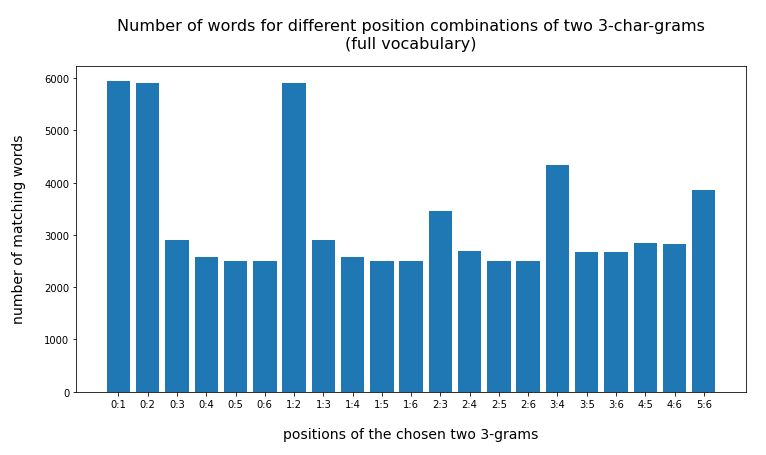The absolute numbers are much too big. But this plot already gives a clear indication that larger distances between the two 3-char-grams are better to limit the size of the result set. When we use the reduced vocabulary slice (with words shorter than 13 letters) we get

{'0:1': 1305, '0:2': 1277, '0:3': 143, '0:4': 48, '0:5': 20, '0:6': 24, '1:2': 1279, '1:3': 143, '1:4': 48, '1:5': 20, '1:6': 24, '2:3': 450, '2:4': 125, '2:5': 23, '2:6': 31, '3:4': 634, '3:5': 58, '3:6': 55, '4:5': 76, '4:6': 72, '5:6': 263}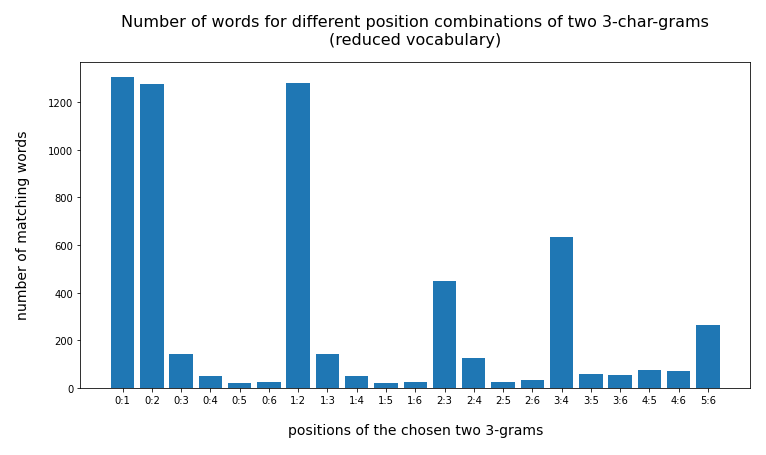For some combinations the resulting hit list is much shorter (< 50)! And the effect of some distance between the chosen char-grams gets much more pronounced.

Corresponding data for the words "löwenzahn" and "kellertür" confirm the tendency:

Test-word "löwenzahn"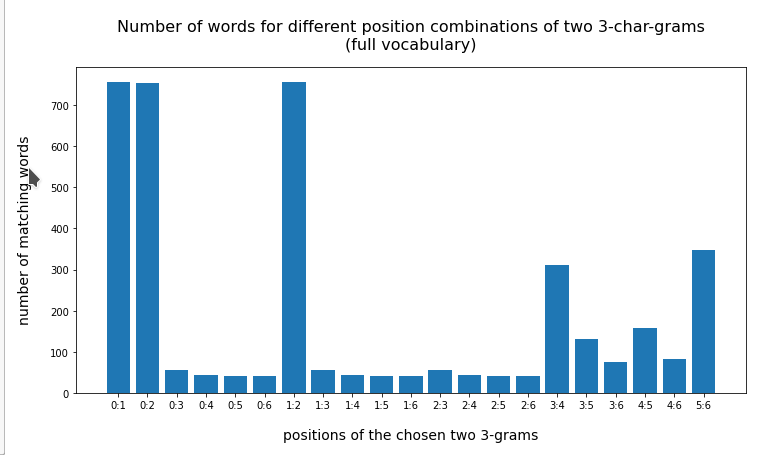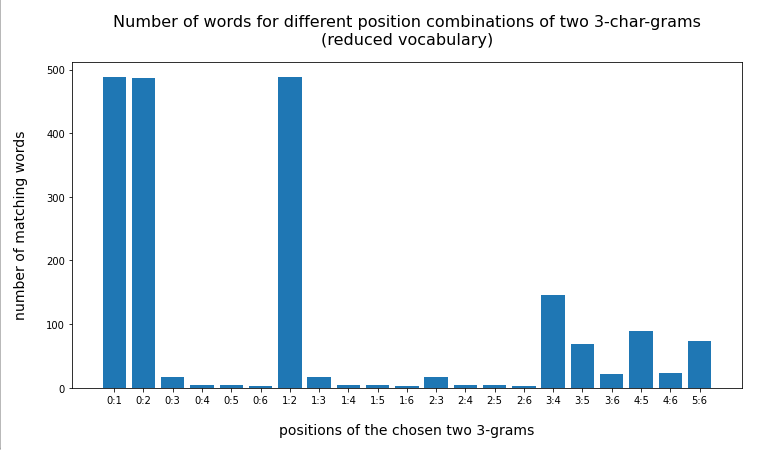Watch the lower numbers along the y-scale!

Test-token "kellertür"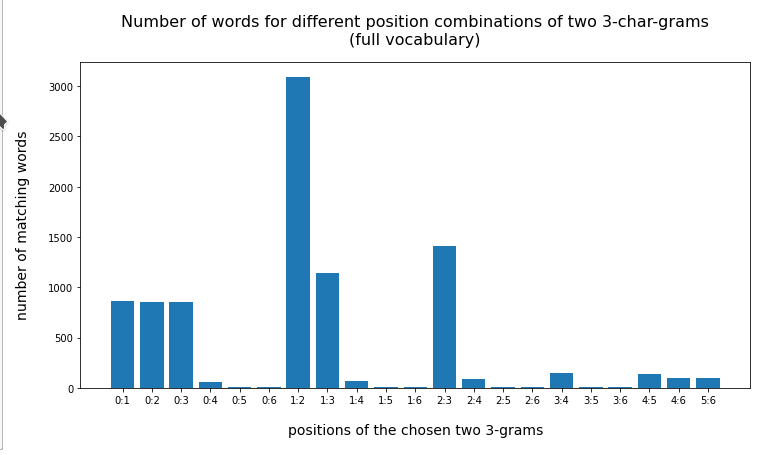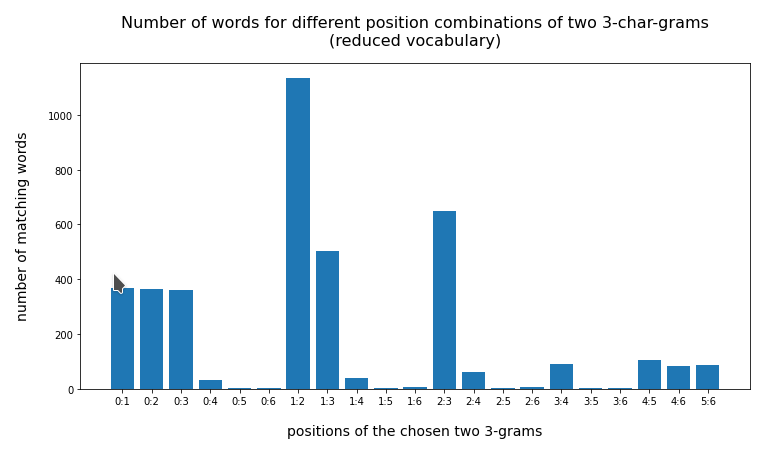Using the information about the word length for optimization

On average the above numbers are still too big for a later detailed comparative analysis with our test token - even on the reduced vocabulary. We expect an improvement by including the length information. What numbers do we get when we use a list with words having exactly the same length as the test-word?

You find the results below:

Test-token "eisenbahn"

{'0:1': 158, '0:2': 155, '0:3': 16, '0:4': 6, '0:5': 1, '0:6': 3, '1:2': 155, '1:3': 16, '1:4': 6, '1:5': 1, '1:6': 3, '2:3': 83, '2:4': 37, '2:5': 3, '2:6': 9, '3:4': 182, '3:5': 17, '3:6': 17, '4:5': 22, '4:6': 22, '5:6': 109}

Test-token "löwenzahn"

{'0:1': 94, '0:2': 94, '0:3': 3, '0:4': 2, '0:5': 2, '0:6': 1, '1:2': 94, '1:3': 3, '1:4': 2, '1:5': 2, '1:6': 1, '2:3': 3, '2:4': 2, '2:5': 2, '2:6': 1, '3:4': 54, '3:5': 43, '3:6': 13, '4:5': 59, '4:6': 14, '5:6': 46}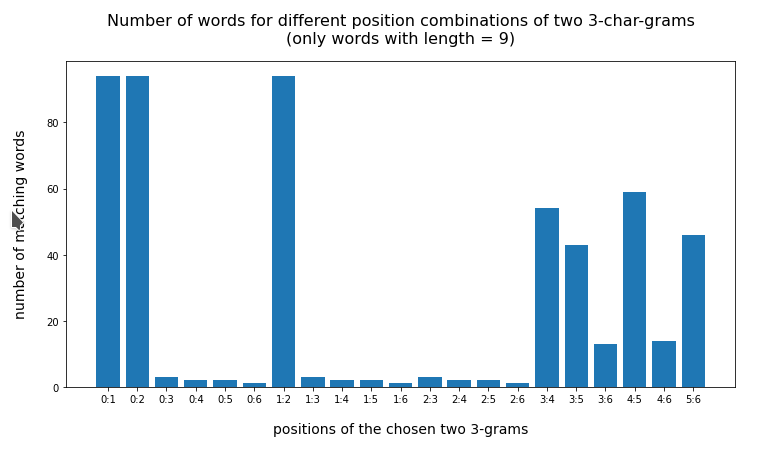Test-token "kellertür"

{'0:1': 14, '0:2': 13, '0:3': 13, '0:4': 5, '0:5': 1, '0:6': 1, '1:2': 61, '1:3': 24, '1:4': 5, '1:5': 1, '1:6': 2, '2:3': 36, '2:4': 8, '2:5': 1, '2:6': 3, '3:4': 12, '3:5': 1, '3:6': 1, '4:5': 17, '4:6': 17, '5:6': 17}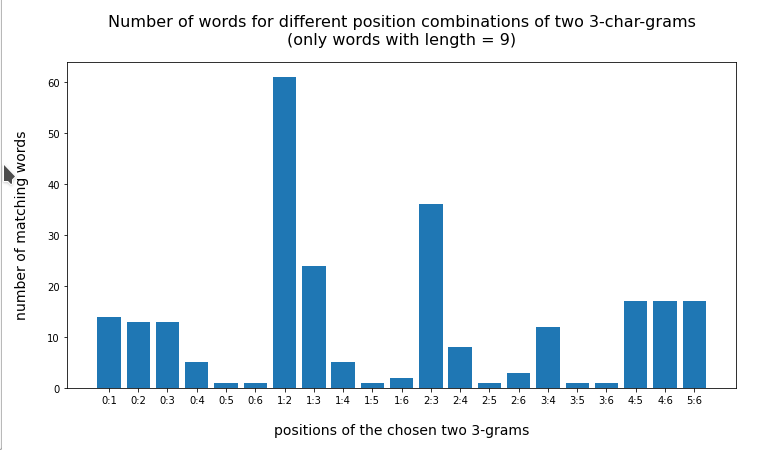For an even shorter word like "vogelart" and "nashorn" two 3-char-grams cover almost all of the word. But even here the number of hits is largest for neighboring 3-char-grams:

Test-word "vogelart" (8 letters)

{'val_0': 'vog', 'val_1': 'oge', 'val_2': 'gel', 'val_3': 'ela', 'val_4': 'lar', 'val_5': 'art', 'val_6': 'rt'}
{'0:1': 22, '0:2': 22, '0:3': 1, '0:4': 1, '0:5': 1, '1:2': 23, '1:3': 1, '1:4': 1, '1:5': 2, '2:3': 10, '2:4': 6, '2:5': 5, '3:4': 19, '3:5': 15, '4:5': 24}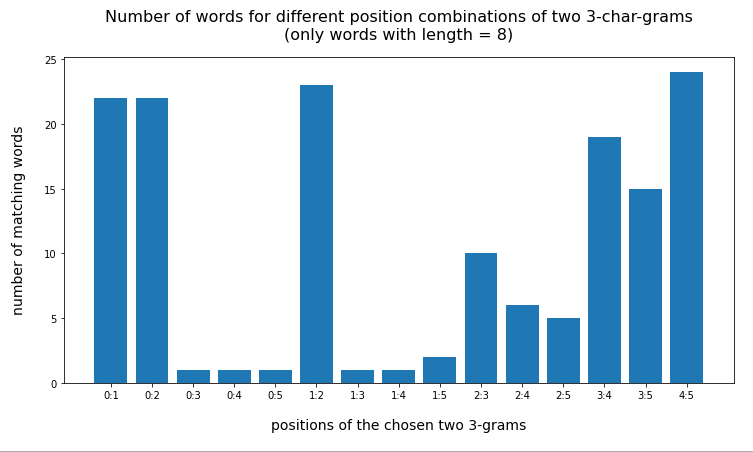Test-word "nashorn" (7 letters)

{'val_0': 'nas', 'val_1': 'ash', 'val_2': 'sho', 'val_3': 'hor', 'val_4': 'orn', 'val_5': 'rn', 'val_6': 'n'}
{'0:1': 1, '0:2': 1, '0:3': 1, '0:4': 1, '1:2': 1, '1:3': 1, '1:4': 1, '2:3': 3, '2:4': 2, '3:4': 26}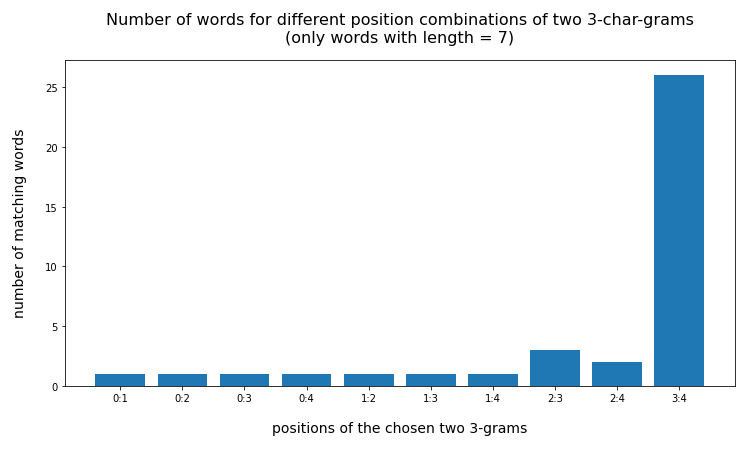So, as an intermediate result I would say:

• Our naive idea about using 3-char-grams with some distance between them is pretty well confirmed for relatively small words with a length below 9 letters and two 3-char-grams.
• We should use the length information about a test-word or token in addition to diminish the list of reasonably matching words!

Code to investigate 3-char-gram combinations for words with more than 9 letters

Let us now turn to longer words. Here we face a problem: The number of possibilities to choose three 3-char-grams at different positions explodes with word-length (simple combinatorics leading to the binomial coefficient). It is even difficult to present results graphically. Therefore, I had to restrict myself to gram-combinations with some reasonable distance from the beginning.

The following code does not exclude anything and leads to problematic plots:

# Hits for two 3-grams distributed over a 13-letter word
# ******************************************************
b_full_vocab  = False # operate on the full vocabulary
#b_full_vocab  = True # operate on the full vocabulary

#word  = "nachtwache"             # 10
#word  = "morgennebel"            # 11
#word  = "generalmajor"           # 12
#word  = "gebirgskette"           # 12
#word  = "fussballfans"           # 12
#word  = "naturforscher"          # 13
#word  = "frühjahrsputz"          # 13
#word  = "marinetaucher"          # 13
#word  = "autobahnkreuz"          # 13
word  = "generaldebatte"         # 14
#word  = "eiskunstläufer"         # 14
#word  = "gastwirtschaft"         # 14
#word  = "vergnügungspark"        # 15
#word  = "zauberkuenstler"        # 15
#word  = "abfallentsorgung"       # 16
#word  = "musikveranstaltung"     # 18
#word  = "sicherheitsexperte"     # 18
#word  = "literaturwissenschaft"  # 21
#word  = "veranstaltungskalender" # 23

len_w = len(word)
print(len_w, math.floor(len_w/2))

d_col = { "col_0": "gram_2",   "col_1": "gram_3",   "col_2": "gram_4",   "col_3": "gram_5",
"col_4": "gram_6",   "col_5": "gram_7",   "col_6": "gram_8",   "col_7": "gram_9",
"col_8": "gram_10",  "col_9": "gram_11",  "col_10": "gram_12", "col_11": "gram_13",
"col_12": "gram_14", "col_13": "gram_15", "col_14": "gram_16", "col_15": "gram_17",
"col_16": "gram_18", "col_17": "gram_19", "col_18": "gram_20", "col_19": "gram_21"
}
d_val = {}

ind_max = len_w - 2

for i in range(0,ind_max):
key_val  = "val_" + str(i)
sl_start = i
sl_stop  = sl_start + 3
val = word[sl_start:sl_stop]
d_val[key_val] = val
print(d_val)

li_cols =  # list of cols to display in a final dataframe

d_num = {}
li_permut = []

# prepare short
length  = len_w
mil = min_len - 1
mal = max_len + 1
b_exact_length = True
if b_exact_length:
dfw_short = dfw_uml.loc[(dfw_uml.lower.str.len() == length)]
else:
dfw_short = dfw_uml.loc[(dfw_uml.lower.str.len() > mil) & (dfw_uml.lower.str.len() < mal)]
dfw_short = dfw_short.iloc[:, 2:26]
print(len(dfw_short))

# find matching words for all position combinations
# ~~~~~~~~~~~~~~~~~~~~~~~~~~~~~~~~~~~~~~~~~~~~~~~~~
for i in range(0,ind_max):
for j in range(0,ind_max):
for k in range(0,ind_max):
if (i,j,k) in li_permut or (i==j or j==k or i==k):
continue
else:
col_name1 = "col_" + str(i)
val_name1 = "val_" + str(i)
col1 = d_col[col_name1]
val1 = d_val[val_name1]
col_name2 = "col_" + str(j)
val_name2 = "val_" + str(j)
col2 = d_col[col_name2]
val2 = d_val[val_name2]
col_name3 = "col_" + str(k)
val_name3 = "val_" + str(k)
col3 = d_col[col_name3]
val3 = d_val[val_name3]
li_akt_permut = list(itertools.permutations([i, j, k]))
li_permut = li_permut + li_akt_permut
#print("i,j,k = ", i, ":", j, ":", k)
#print(len(li_permut))

# matches ?
if b_full_vocab:
li_ind = dfw_uml.index[  (dfw_uml[col1]==val1)
&    (dfw_uml[col2]==val2)
&    (dfw_uml[col3]==val3)
].tolist()
else:
li_ind = dfw_short.index[(dfw_short[col1]==val1)
&    (dfw_short[col2]==val2)
&    (dfw_short[col3]==val3)
].tolist()

num = len(li_ind)
key = str(i)+':'+str(j)+':'+str(k)
d_num[key] = num
print("length of d_num = ", len(d_num))
print(d_num)

# bar diagram
fig_size = plt.rcParams["figure.figsize"]
fig_size = 15
fig_size = 6
names  = list(d_num.keys())
values = list(d_num.values())
plt.bar(range(len(d_num)), values, tick_label=names)
plt.xlabel("positions of the chosen two 3-grams", fontsize=14, labelpad=18)
plt.ylabel("number of matching words", fontsize=14, labelpad=18)
font_weight = 'bold'
font_weight = 'normal'
if b_full_vocab:
elif  (not b_full_vocab and not b_exact_length):
else:
add_title = "\n(only words with length = " + str(len_w) + ")"

plt.title("Number of words for different position combinations of two 3-char-grams" + add_title,
plt.show()

An example for the word "generaldebatte" (14 letters) gives: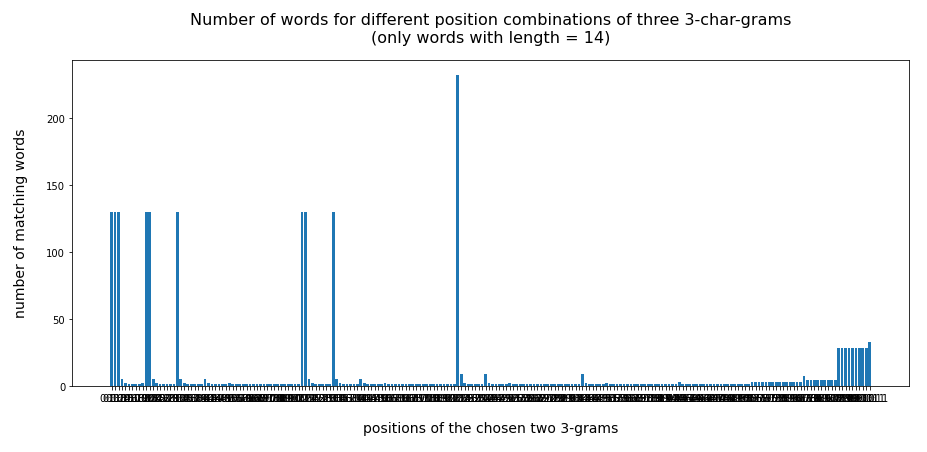A supplemental code that reduces the set of gram position combinations significantly to larger distances could look like this:

# Analysis for 3-char-gram combinations with larger positional distance
# ********************************************************************

hf = math.floor(len_w/2)

d_l={}
for i in range (2,26):
d_l[i] = {}

for key, value in d_num.items():
li_key = key.split(':')
# print(len(li_key))
i = int(li_key)
j = int(li_key)
k = int(li_key)
l1 = int(li_key) - int(li_key)
l2 = int(li_key) - int(li_key)
le = l1 + l2
# print(le)
if (len_w < 12):
bed1 = (l1<=1 or l2<=1)
bed2 = (l1 <=2 or l2 <=2)
bed3 = (((i < hf and j< hf and k< hf) or (i > hf and j> hf and k > hf)))
if (len_w < 15):
bed1 = (l1<=2 or l2<=2)
bed2 = (l1 <=3 or l2 <=3)
bed3 = (((i < hf and j< hf and k< hf) or (i > hf and j> hf and k > hf)))
elif (len_w <18):
bed1 = (l1<=3 or l2<=3)
bed2 = (l1 <=4 or l2 <=4)
bed3 = (((i < hf and j< hf and k< hf) or (i > hf and j> hf and k > hf)))
else:
bed1 = (l1<=3 or l2<=3)
bed2 = (l1 <=4 or l2 <=4)
bed3 = (((i < hf and j< hf and k< hf) or (i > hf and j> hf and k > hf)))

for j in range(2,26):
if le == j:
if value == 0 or bed1 or ( bed2 and bed3) :
continue
else:
d_l[j][key] = value

sum_len = 0
n_p = len_w -2
for j in range(2,n_p):
num = len(d_l[j])
print("len = ", j, " : ", "num = ", num)

print()
print("len_w = ", len_w, " half = ", hf)

if (len_w <= 12):
p_start = hf
elif (len_w < 15):
p_start = hf + 1
elif len_w < 18:
p_start = hf + 2
else:
p_start = hf + 2

# Plotting
# ***********
li_axa = []
m = 0
for i in range(p_start,n_p):
if len(d_l[i]) == 0:
continue
else:
m+=1
print(m)
fig_size = plt.rcParams["figure.figsize"]
fig_size = 12
fig_size = m * 5
fig_b  = plt.figure(2)

for j in range(0, m):

m = 0
for i in range(p_start,n_p):
if len(d_l[i]) == 0:
continue
# bar diagram
names  = list(d_l[i].keys())
values = list(d_l[i].values())
li_axa[m].bar(range(len(d_l[i])), values, tick_label=names)
li_axa[m].set_xlabel("positions of the 3-grams", fontsize=14, labelpad=12)
li_axa[m].set_xticklabels(names, fontsize=12, rotation='vertical')
#font_weight = 'bold'
font_weight = 'normal'
if b_full_vocab:
elif  (not b_full_vocab and not b_exact_length):
add_title = " (reduced vocabulary)"against position-combinations for <em>three</em> 3-char-grams</h1>
else:
add_title = " (word length = " + str(len_w) + ")"

li_axa[m].set_title("total distance = " + str(i) + add_title,
m += 1

fig_b.suptitle("word :  " + word +" (" + str(len_w) +")", fontsize=24,
fontweight='bold', y=0.91)
plt.show()

What are the restrictions? Basically

• we eliminate combinations with 2 neighboring 3-char-grams,
• we eliminate 3-char-grams combinations where all 3-grams are place only on one side of the word - the left or right one,
• we pick only 3-char-grams where the sum of the positional distances between the 3-char-grams is somewat longer than half of the token's length.

We vary these criteria a bit with the word length. In my opinion these criteria should produce plots, only, which show that the number of hits is reasonably small - if our basic approach is of some value.

Number of matching words with more than 9 letters against position-combinations for three 3-char-grams

The following plots cover words of different growing lengths for dataframes reduced to words with exactly the same length as the chosen token. Not too surprising, all of the words are compound words.

**************************

Test-token "musikveranstaltung"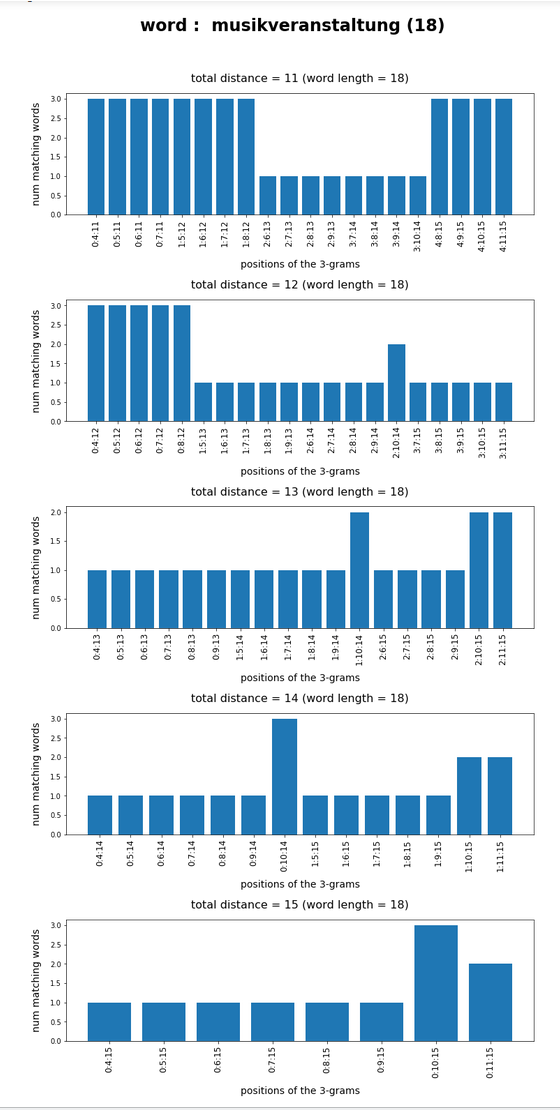Test-token "sicherheitsexperte"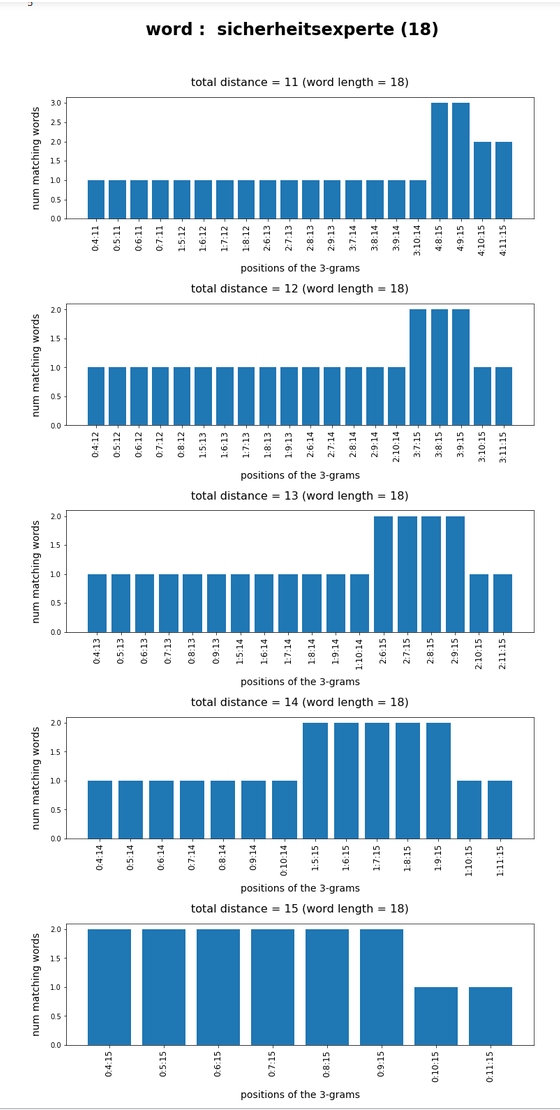**************************

What we see is that whenever we choose 3-char-gram combinations with a relative big positional distance between them and a sum of the two distances ≥ word-length / 2 + 2 the number of matching words ogf the vocabulary is smaller than 10, very often even smaller than 5. The examples "prove" at least that choosing three (correctly written) 3-char-grams with relative big distance within a token lead to small numbers of matching vocabulary words,

Conclusion

One can use a few 3-char-grams within string tokens to find matching vocabulary words via a comparison of the char-grams at their respective position. In this article we have studied how we should choose two or three 3-char-grams within string tokens of length ≤ 9 letters or > 9 letters, respectively, if and when we want to find matching vocabulary words effectively. We found strong indications that the 3-char-grams should be chosen with a relatively big positional distance. To use neighboring 3-char-grams will lead to hit numbers which are too big for a detailed analysis.

In the next post I will have a closer look at the required CPU-time for a word searches in a vocabulary based on 3-char-gram comparisons for a 100,000 string tokens.